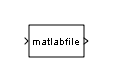# Level-2 MATLAB S-Function

Use Level-2 MATLAB S-function in model

• Library:

•## Description

This block allows you to use a Level-2 MATLAB® S-function (see Write Level-2 MATLAB S-Functions) in a model. To do this, create an instance of this block in the model. Then enter the name of the Level-2 MATLAB S-function in the S-function name field of the block's parameter dialog box.

Note

Use the S-Function block to include a Level-1 MATLAB S-function in a block.

If the Level-2 MATLAB S-function defines any additional parameters, you can enter them in the Parameters field of the block's parameter dialog box. Enter the parameters as MATLAB expressions that evaluate to their values in the order defined by the MATLAB S-function. Use commas to separate each expression.

If a model includes a Level-2 MATLAB S-Function block, and an error occurs in the S-function, the Level-2 MATLAB S-Function block displays MATLAB stack trace information for the error in a dialog box. Click to close the dialog box.

## Parameters

expand all

Specify the name of a MATLAB function that defines the behavior of this block. The MATLAB function must follow the Level-2 standard for writing MATLAB S-functions (see Write Level-2 MATLAB S-Functions for details).

#### Programmatic Use

 Block Parameter: `FunctionName` Type: character vector Values: `'matlabfile'` | S-Function name Default: `'matlabfile'`

Specify values of parameters for this block.

#### Programmatic Use

 Block Parameter: `Parameters` Type: character vector Values: values of block parameters Default: `' '`

## Block Characteristics

 Data Types `Booleana` | `doublea` | `fixed pointa` | `integera` | `singlea` Direct Feedthrough `yes` Multidimensional Signals `yes` Variable-Size Signals `yes` Zero-Crossing Detection `no` a Level-1 MATLAB S-functions support only the double data type. Level-2 MATLAB S-functions support all data types that Simulink supports.

## Version History

Introduced in R2010b